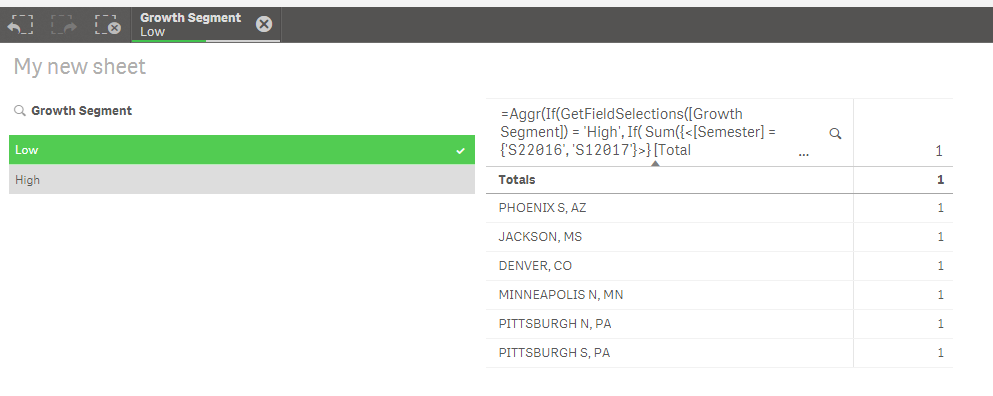New to Qlik Sense

Discussion board where members can get started with Qlik Sense.

Announcements
QlikWorld 2020: Join us May 11 - 14, 2020 in Phoenix, AZ. Register early and save \$400. Learn MoreMVP

Re: second part of if condition not working

Are you sure there are Territory Name which meet this criteria?

Sum({<[Semester] = {'S22016', 'S12017'}>} [Total Sales])/Sum({<[Semester] = {'S22015', 'S12016'}>} [Total Sales]) <

Sum(TOTAL {<[Semester] = {'S22016', 'S12017'}>} [Total Sales])/Sum(TOTAL {<[Semester] = {'S22015', 'S12016'}>} [Total Sales])

Contributor

Re: second part of if condition not working

Yes. Also, I am comparing it with the average sales growth so it should have the values. I have shared a sample data set in this thread if you can take a look. Thanks for your help though.MVP

Re: second part of if condition not working

I saw the sample dataset, but how is this loaded into your dashboard? Everything is a field or do you use CrossTable Load?

Contributor

Re: second part of if condition not working

I did not use crosstable load. I imported the data directly using Add Data.

HighlightedMVP

Re: second part of if condition not working

This seems to have worked

=Aggr(If(GetFieldSelections([Growth Segment]) = 'High',

If(

Sum({<[Semester] = {'S22016', 'S12017'}>} [Total Sales])/Sum({<[Semester] = {'S22015', 'S12016'}>} [Total Sales]) >

Sum(TOTAL {<[Semester] = {'S22016', 'S12017'}>} [Total Sales])/Sum(TOTAL {<[Semester] = {'S22015', 'S12016'}>} [Total Sales]), [Territory Name]),

If(

Sum({<[Semester] = {'S22016', 'S12017'}>} [Total Sales])/Sum({<[Semester] = {'S22015', 'S12016'}>} [Total Sales]) <

Sum(TOTAL {<[Semester] = {'S22016', 'S12017'}>} [Total Sales])/Sum(TOTAL {<[Semester] = {'S22015', 'S12016'}>} [Total Sales]), [Territory Name])), [Territory Name])Here Growth Segment is a field from an Island table

Contributor

Re: second part of if condition not working

Wow! It's working.. Thanks a lot. I still need to understand the code but it's working. Thanks a ton!MVP

Re: second part of if condition not working

You should have a much easier time understanding this expression because at least to me, it looks much easier to read then the one you initially had....

Contributor

Re: second part of if condition not working

Yes, I understand. The thing is I am pretty new to Qliksense and haven't explored the set analysis as yet.

Contributor

Re: second part of if condition not working

If I use the similar code in a table it again has the same problem. I have used growth segments as dimensions and the code calculation as a measure. Following code is used:

sum(Aggr(If([Segments] = 'High',

If(

Sum({<[Semester] = {'S22016', 'S12017'}>} [Total Sales])/Sum({<[Semester] = {'S22015', 'S12016'}>} [Total Sales]) >

Sum(TOTAL {<[Semester] = {'S22016', 'S12017'}>} [Total Sales])/Sum(TOTAL {<[Semester] = {'S22015', 'S12016'}>} [Total Sales]), count(distinct [Territory Name])),

If(

Sum({<[Semester] = {'S22016', 'S12017'}>} [Total Sales])/Sum({<[Semester] = {'S22015', 'S12016'}>} [Total Sales]) <

Sum(TOTAL {<[Semester] = {'S22016', 'S12017'}>} [Total Sales])/Sum(TOTAL {<[Semester] = {'S22015', 'S12016'}>} [Total Sales]), count(distinct [Territory Name]))), [Territory Name]))

It shows a number for High but not for Low.. Can you please let me know why this keeps on happening ? So I can get it right by myself the next time.. Thanks.

Best Regards,MVP

Re: second part of if condition not working

You need to add Growth Segment to your Aggr() function

Sum(Aggr(If([Growth Segment] = 'High',

If(

Sum({<[Semester] = {'S22016', 'S12017'}>} [Total Sales])/Sum({<[Semester] = {'S22015', 'S12016'}>} [Total Sales]) >

Sum(TOTAL {<[Semester] = {'S22016', 'S12017'}>} [Total Sales])/Sum(TOTAL {<[Semester] = {'S22015', 'S12016'}>} [Total Sales]), count(distinct [Territory Name])),

If(

Sum({<[Semester] = {'S22016', 'S12017'}>} [Total Sales])/Sum({<[Semester] = {'S22015', 'S12016'}>} [Total Sales]) <

Sum(TOTAL {<[Semester] = {'S22016', 'S12017'}>} [Total Sales])/Sum(TOTAL {<[Semester] = {'S22015', 'S12016'}>} [Total Sales]), count(distinct [Territory Name]))), [Territory Name], [Growth Segment]))